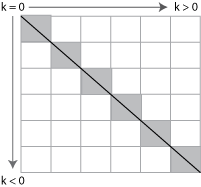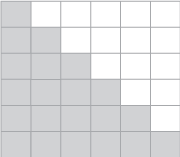# tril

## 语法

``L = tril(A)``
``L = tril(A,k)``

## 说明

``L = tril(A)` 返回矩阵 `A` 的下三角部分。`
``L = tril(A,k)` 返回 `A` 的第 k 个对角线上以及该对角线下方的元素。`

## 示例

`A = ones(4)`
```A = 4×4 1 1 1 1 1 1 1 1 1 1 1 1 1 1 1 1 ```
`B = tril(A)`
```B = 4×4 1 0 0 0 1 1 0 0 1 1 1 0 1 1 1 1 ```

`C = tril(A,-1)`
```C = 4×4 0 0 0 0 1 0 0 0 1 1 0 0 1 1 1 0 ```

## 输入参数## 详细信息

### 下三角矩阵